#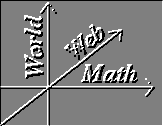Vector Calculus Notation

Mathematical notation is designed to create the correct ideas in the mind of the reader. It is deliberately ambiguous and incomplete; indeed, it is almost meaningless to all other readers.
-- Poppelier, Herwijnen and Rowley, "Standard dtd's and scientific publishing"

As the following table demonstrates, the notations used in vector calculus are myriad. Indeed, this table is not exhaustive.

For the sake of uniformity, these pages will in general use bold letters for vectors and unit vectors, such as v for a vector and i for a unit vector.
Symbol Definition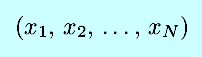N-tuple of real numbersSpace of all N-tuples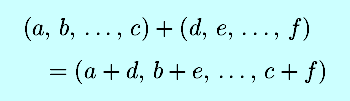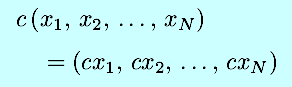Scalar multiplication of an N-tupleThe origin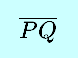the line segment between P and Q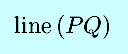the line through P and QA vector variableThe zero vectorThe magnitude of a vector variable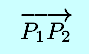Displacement vector from P1 to P2Unit vector in the direction of the positive x-axisUnit vector in the direction of the positive y-axis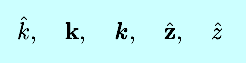Unit vector in the direction of the positive z-axis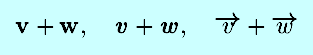Vector negatition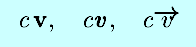Scalar multiplication of a vector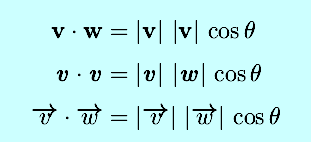Geometric definition of dot product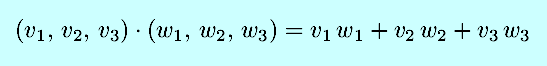Algebraic definition of dot product (N=3)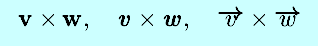Cross productDistance from z-axis in cylindrical coordinates

Vector Calculus Index | World Web Math Categories Page

watko@athena.mit.edu